# Difference between revisions of "Euler-Fourier formulas"

Formulas for the coefficients of a Fourier series.

Ifis a function of period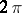defined on the real line and if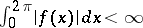, then the formulas are: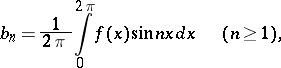and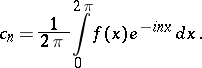The formal series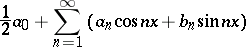and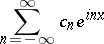are both called the Fourier series of. They have the same partial sums: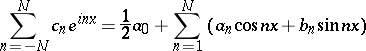for alland all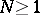. The coefficient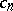is also denoted by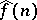.

How to Cite This Entry:
Euler-Fourier formulas. Encyclopedia of Mathematics. URL: http://www.encyclopediaofmath.org/index.php?title=Euler-Fourier_formulas&oldid=12665